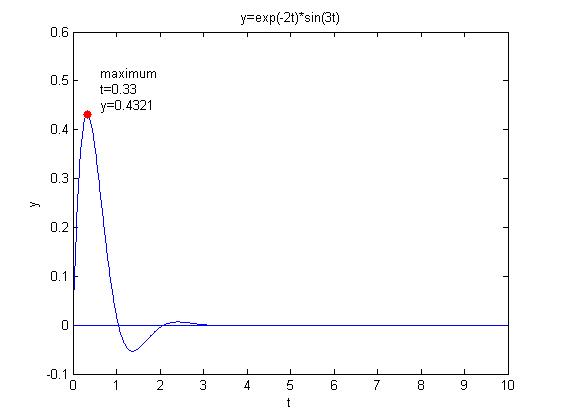• Matlab的优势在于向量...　字符串数组我通常会选择应用cell格式保存，下面的分析也是建立在这个前提下。 　 　【1】strcmp() 函数 　strcmp() 函数的基本功能是比较两个字符串是否相等，其基本用法是： 1 T...

Matlab的优势在于向量操作，字符串操作往往费时费力，但是如果能充分利用Matlab自带的一些函数，也可以取得不错的效果。下面就介绍一下字符串数组查找的小技巧。
字符串数组我通常会选择应用cell格式保存，下面的分析也是建立在这个前提下。

【1】 strcmp() 函数
strcmp() 函数的基本功能是比较两个字符串是否相等，其基本用法是：

1 TF = strcmp(s1,s2);

但是，如果我们要查找字符串数组中等于某字符串的索引时，该如果操作？strcmp() 函数也提供了这个功能，用法相同：

1 TF = strcmp(s1,s2);

其中，s1是字符串数组，s2是字符串，返回值为逻辑类型，大小与字符串数组s1相同；
【2】 strfind() 函数

strcmp() 函数比较的是两个字符串是否相等。但是如果想查找字符串数组中包含某字符串的记录时，我们就可以选择strfind() 函数。

1 k = strfind(str,pattern);

输出结果k表示pattern在str中出现的位置，若不出现，则返回[]。比如：

1 S = ‘Find the starting indices of the pattern string’;
2 k = strfind(S, ‘in’)
3 k =
4     2    15    19    45

上面是strfind() 函数的基本功能，但是如果查找字符串数组中包含某字符串的索引时，strfind() 函数也可以大显身手，返回和字符串数组相同大小的cell类型数据，每个cell为字符串出现位置，向量类型，比如：

1 cstr = {‘How much wood would a woodchuck’; ‘if a woodchuck could chuck wood?’};
2 idx = strfind(cstr, ‘wood’)
3 idx =
4     [1*2 double]
5     [1*2 double]
6 Idx{:,:}
7 ans =
8     10    23
9 ans =
10     6    28

【3】 一个问题：有一个字符串数组cstr1，要找到包含字符串str2的索引，该如果操作？
一个直接的答案是：

1 idx = strfind(cstr1,str2);
2 finger(length(idx),1) = 1==0;
3 for i = 1:length(idx)
4     if ~isempty(idx(i))
5         finger(i) = 1==1;
6     end
7 end

思路是正确的，但是代码量有点大，需要7行，而且速度较慢。其实要实现这个功能，1行代码足矣。如下：

1 finger = ~cellfun(@isempty, strfind(cstr1,str2));

上面的代码还有另外一种写法，

1 finger = cellfun(@(x) ~isempty(strfind(x,str2)), cstr1);

三种方法输出结果是一样的，但是第2种方法速度最快，第1种方法次之，第3种方法最慢。大家可以进行测试下。

转载于:https://www.cnblogs.com/anzhiwu815/p/5885097.html
展开全文• ## MATLAB字符串数组

千次阅读 2020-07-03 10:41:23
MATLAB字符串数组 基本规则 (1)所有字符串都用单引号（英文状态下输入）括起来； (2)将字符串当作一个行向量，每个元素对应一个字符，其标识方法和数值向量相同。 (3)size指令获得串数组的大小。串中的每一个字符，...
MATLAB字符串数组
基本规则
(1)所有字符串都用单引号（英文状态下输入）括起来；
(2)将字符串当作一个行向量，每个元素对应一个字符，其标识方法和数值向量相同。
(3)size指令获得串数组的大小。串中的每一个字符，包括中英文字符、空格、标点都算一个元素。
(4)字符串中的字符以ASCⅡ码形式存储，因而区分大小写。
(5)可建立多行字符串，形成字符串矩阵。
例如
String1='welcome to matlab!'		%单引号括起来
String1 =
'welcome to matlab!'

String1='9527 welcome to matlab !! '
size(String1)

String1 =

'9527 welcome to matlab !! '		%包含数字，字母，标点符号，空格

ans =

1    26					%长为26

ch=['abcdef';'123456']   	 %建立多行字符串，形成字符串矩阵
ch(2,3)

ch =

2×6 char 数组				%建立的2行6列的字符串矩阵

'abcdef'
'123456'

ans =					%第2行3列的元素为'3'

'3'

abs和double指令可以显示字符数组对应的ASCII码数值数组。char指令则可以把ASCII码数值数组转换成字符数组。
s1='MATLAB';
a=abs(s1)			%将字符数组转化为ascll码值数组
b=double(s1)
char(a)				%将ascll码值数组转化为字符数组

%输出如下
a =

77    65    84    76    65    66

b =

77    65    84    76    65    66

ans =

'MATLAB'

例题：建立一个字符串向量，然后对该向量做如下处理：
(1)取第1～5个字符组成的子字符串。
(2)将字符串倒过来重新排列。
(3)将字符串中的小写字母变成相应的大写字母，其余字符不变。
(4)统计字符串中小写字母的个数。
clc;
ch='ABc123d4e56Fg9' ;
subch=ch(1:5)           %取子字符串
revch=ch(end:-1:1)       %将字符串倒排
k=find(ch>='a' & ch<='z')  %找小写字母的位置
ch(k)=ch(k)-('a'-'A')   	 %小写字母变成大写
length(k)      			 %统计小写字母的个数

对于上述例题的解释
1.找出的是小写字母的位置，结果输出为3 7 9 13，即在第3 7 9  13的位置是小写字母
k=find(ch>='a' & ch<='z')  %找小写字母的位置

2.提出小写字母，输出结果为ans = ‘CDEG’
ch(k)		%将小写字母位置上的小写字母提出

ch(k)-('a'-'A')
%将找出的小写字母，ascll码值减去32（即大小写字母的ascll码值差），转换成大写字母

当两个字符串数组进行关系比较时，是将其对应位置的字符的ascll码值进行比较
字符处理函数及其含义

函数名
含义

setstr
将ASCII码值转换成字符

char
同上，将代替setstr

mat2str
将矩阵转换成字符串

int2str
将整数转换成字符串

num2str
将数值转换成字符串

str2num
将字符串转换成数值

strcat
用于字符串的连接

abs
将字符转换成ASCII码

eval
把字符串的内容作为对应的命令来执行

findstr(s1,s2)
返回短字符串在长字符串中的开始位置

strrep(s1,s2,s3)
将字符串s1中的所有子字符串s2替换为字符串s3

strcmp(s1,s2)
用来比较字符串s1和s2是否相等，如果相等，返回结果为1，否则 返回0

strncmp(s1,s2,n)
用来比较两个字符串前n个字符是否相等，如果相等，返回1， 否则返回0

strcmpi(s1,s2)
在忽略字母大小写前提下，比较字符串s1和s2是否相等，如果相 等，返回1，否则返回0

strncmpi(s1,s2,n)
在忽略字符串大小写前提下，比较两个字符串前n个字符是否 相等，如果相等，返回1，否则返回0

具体参数内容可输入help进行查看


展开全文• 字符串数组matlab中尤其重要，很多字符串之间的连接通过简单的字符串操作就可以实现。 字符串的创建方式：在命令窗口中，先把待建立的字符放在“单引号对”中，再按enter。注意，单引号对必须是英文状态下输入，...
字符串数组在matlab中尤其重要，很多字符串之间的连接通过简单的字符串操作就可以实现。
字符串的创建方式：在命令窗口中，先把待建立的字符放在“单引号对”中，再按enter。注意，单引号对必须是英文状态下输入，单引号对的作用时matlab用来识别送来内容“身份”（是变量名、数字等）所必须的。
实例1：数值量与字符串的区别
>> a=12345.6789

a =

1.2346e+04

>> class(a)   %判别数据类别

ans =

double

>> a_s=size(a)     %确定数组的规模

a_s =

1     1

>> b='S'

b =

S

>> class(b)

ans =

char

>> b_s=size(b)

b_s =

1     1

>> whos   %列出工作内存中的变量细节
Name      Size            Bytes  Class     Attributes

a         1x1                 8  double
a_s       1x2                16  double
ans       1x4                 8  char
b         1x1                 2  char
b_s       1x2                16  double             
实例2，串的基本属性、标识和简单操作
1）创建串数组：直接键入，赋给变量即可。
>> a='This is an example.'

a =

This is an example.
2）串数组的大小：在以上赋值后，变量a就是一个串数组，串数组中每个字符（每个都算）占据一个元素位。串数组的大小可以用下面的命令获得：
>> size(a)

ans =

1    19

3）中文字符串数组：键入时注意单引号对仍然要在英文状态下输入，其他没有什么。
>> A='这是算例。'

A =

这是算例。
4）字符串的相互连接：字符串的这个功能应该会很有用！
>> ab=[A(1:4),'a.1-2',A(5)] %字符串数组也能援引

ab =

这是算例a.1-2。
实例3，实现数值向字符串转换的函数int2str,num2str。
1）int2str把整数数组转换成串数组（非整数将被四舍五入之后再进行转换）
>> A=eye(2,4);
>> A_str=int2str(A) %转换成2*10串数组。

A_str =

1  0  0  0
0  1  0  0

>> size(A) %数值数组的规模很容易理解

ans =

2     4

>> size(A_str)  %字符串数组之所以显示每行10个，是因为每两个数字字符之间的有两个间隔。

ans =

2    10
字符串数组数字之间两个间隔，可以通过查看每一个字符串的元素得知。
>> A_str(1,1)

ans =

1

>> A_str(1,2)

ans =

>> A_str(1,3)

ans =

>> A_str(1,4)

ans =

0

>> A_str(1,5)

ans =

>> A_str(1,6)

ans =

>> A_str(1,7)

ans =

0

>> A_str(1,8)

ans =

>> A_str(1,9)

ans =

>> A_str(1,10)

ans =

0
2）num2str把非整数数组转换为串数组（常用于图形中数据点的标识）

>> rng default %默认全局随机流操控，rng的用法后续会专门研究一下
>> B=rand(2,4); %生成数值矩阵
>> B3=num2str(B,3) %保持3位有效数字，转换为串

B3 =

0.815     0.127     0.632     0.278
0.906     0.913    0.0975     0.547
实例4，在matlab中计算生成的图形上标出图名和最大值点坐标
>> clear
>> a=2; %设置衰减系数
>> w=3; %设置振荡频率
>> t=0:0.01:10;
>> y=exp(-a*t).*sin(w*t); %计算函数值，产生函数数组
>> [y_max,i_max]=max(y); %找最大值元素位置，从下面结果可以看出，y_max是对应的y值，而i_max则是以0.01为步长对应的位置

y_max =

0.4321

i_max = %最大值对应的是以0.01为步长的第34个位置

34

>> t(i_max)  %通过位置可以计算出实际的x坐标

ans =

0.3300

>> t_text=['t=',num2str(t(i_max))] %将横坐标数值转换为字符串

t_text =

t=0.33

>> t_text=['t=',num2str(t(i_max))];
>> y_text=['y=',num2str(y_max)];  %将纵坐标数值转换为字符串
>> max_text=char('maximum',t_text,y_text); %生成标志最大值点的三行字符串
>> tit=['y=exp(-',num2str(a),'t)*sin(',num2str(w),'t)'];    %生成图名的标题
>> plot(t,zeros(size(t)),'k') %画出纵坐标为0的基准线，黑色
>> plot(t,zeros(size(t)))
>> hold on
>> plot(t,y,'b')
>> plot(t(i_max),y_max,'r.','MarkerSize',20) %用大红点标出最大值点
>> text(t(i_max)+0.3,y_max+0.05,max_text) %在图上书写最大值点的数据值，+0.3和+0.05的目的是将文字偏置放置
>> title(tit),xlabel('t'),ylabel('y')
>> hold off展开全文• 比如一个数组a a(1)='bob' a(2)='ryan' a(3)='richard' 请教如果在matlab里面写 a={'bob','ryan','richard'} a(1)='bob' a(2))='ryan' a(3)='richard'
比如一个数组a
a(1)='bob'
a(2)='ryan'
a(3)='richard'
请教如果在matlab里面写

a={'bob','ryan','richard'}
a(1)='bob'
a(2))='ryan'
a(3)='richard'

展开全文• 还是基于自己毕设的实战，这里就用自己的例子：%这里想要建立一个元素类似于x1,x2,x3,...,xn的数组/向量/一维矩阵，但是后缀数字来源于excel表 X = []; %建立空矩阵，无论维度 Xnum = xlsread("结果.xlsx"...
• 类似于字符数组，不过Matlab字符串是用单引号括起来的字符序列。也是以ASCII码形式存储。 >> str='LuYihang'; >> str(3:6) ans = 'Yiha' 注意：若字符串中的字符含有单引号，则该单引号字符要用两个...
• ## Matlab字符串比较

万次阅读 2018-04-26 14:23:02
http://www.cnblogs.com/anzhiwu815/p/5885097.html　　Matlab的优势在于向量操作，字符串操作...　字符串数组我通常会选择应用cell格式保存，下面的分析也是建立在这个前提下。　【1】 strcmp() 函数　strcmp()...str
• ## Matlab：单元数组

千次阅读 2019-10-23 20:16:07
例如：复数向量、实数矩阵、单元数组、结构数组、文本字符串数组等。 2、单元数组的创建： 1）可以通过对单元数组的赋值来创建单元数组，也可以对单元数组的预分配来创建。 （常见的创建单元空矩阵的语句有：C=...
• 什么叫多维数组2、多维数组建立3、算术运算4、关系运算符5、各类函数find（）函数求向量的最大值和最小值求和与求积平均值与中值二、字符数组字符数组创建字符判断字符串三、元胞数组 前言 本文为matlab学习数组...
• 示例：博主想建立一个1行21列的数组，这21个元素都是”pulse error“字符串： characteristic=repelem("pulse error",21) 补充说明，1、如果用单引号，则构建出来的是字符： characteristic2=repelem(‘pulse ...
• 目录 一、字符串处理函数 二、字符串规则 2.1所有字符串都用单引号（英文状态下输入）括起来；...2.5可建立多行字符串，形成字符串矩阵。 三、指令Demo 一、字符串处理函数 函数名称 详情 setst...
• %这里想要建立一个元素类似于x1,x2,x3,...,xn的数组/向量/一维矩阵，但是后缀数字来源于excel表 X = []; %建立空矩阵，无论维度 Xnum = xlsread("结果.xlsx","sheet2"); %从表“结果.xlsx”的工作簿“sheet2"中读取...
• eval的功能简单来说就是可以把字符串当做命令来执行。经常用于循环当中，特别是有些变量的名字中含有有规律的数字。 二、{ } 大括号，用于cell型的数组（就是前面讲的单元数组）的分配或引用。 比如 a{3,3}=‘china...
• 第四章 元胞数组字符串数组 和结构数组;4.1 元胞数组; 4.1.1元胞数组的建立 组成元胞数组的内容可以是任意类型的数据因此建立元胞数组之前可以先创建相应的数据 1直接赋值法建立元胞数组 建立元胞数组需要使用运算符...
• ## Matlab 快速入门

千次阅读 2019-06-19 09:17:33
文章目录1 MATLAB 产品描述1.1 数学、图形与编程1.2 规模、集成与部署1.3 关键特性2 MATLAB 界面基础知识3 矩阵与数组3.1 数组建立3.2 矩阵和数组运算3.3 数组的连接3.4 复数的表示4 数组的索引5 工作空间变量6 ...
• 例如单元数组、实数矩阵、文本字符串数组、结构数组以及其他复数向量等。 3.16.1 单元数组的创建 可以通过对单元数组的赋值来创建单元数组，也可以对单元数组的预分配来创建。对于单元数组预分配的书写格式为： C=...
• 语法知识 ...例如单元数组、实数矩阵、文本字符串数组、结构数组以及其他复数向量等。 3.16.1 单元数组的创建 C=cell(n) 建立n阶单元空方阵 C=cell（m，n） 建立m行n列的单元空矩阵 C=cell（size(A))...
• Matlab刻意训练 ...例如单元数组、实数矩阵、文本字符串数组、结构数组以及其他复数向量等。 创建 C=cell(n) %建立n阶单元空方阵 C=cell(m,n) %建立m行n列单元空矩阵 C=cell(size(A))...
• MATLAB建立在向量、数组和矩阵的基础 矩阵是MATLAB的核心 ​ MATLAB中变量的命名规则是： （1）变量名必须是不含空格的单个词； （2）变量名区分大小写； （3）变量名最多不超过19个字符； （4）变量名必须以字母打...
• 本书用两章篇幅专述任意高维双精度数值数组、字符串数组、元胞数组和构架数组等四种基本内建数据类型；用九章篇幅分别讲述MATLAB5.3的数值计算、符号计算、函数和数据可视、面向对象编程、GUI交互操作界面设计、EXE...
• matlab建立了14种基本数据结构，每种都以数组形式出现。这些数组最小为零维数组，并能扩展成n维数组，矩阵则被为二维数组。 1.字符数组：用单引号括起来的字符串或称为字符数组。 2.单元数组：可以通过对单元数组的...
• 统计姓名和电子邮箱，建立字符串数组；识别电子邮箱的服务商；统计各电子邮箱的使用人数；将各电子邮箱按照使用人数从多到少排序制成排行榜；发送邮件至邮箱。
• 第二讲 matlab7.0程序设计基础 matlab语言称为第四代编程语言程序简洁可读性很强而且调试十分容易 是matlab重要组成部分 2.1常量与变量 特殊常量 一般变量 2.2数组 2.2.1建立数组 2.2.2引用数组 2.2.3细胞数组与结构...
• MATLAB7.0支持15种基本的数据类型每一种类型的数据都是以矩阵或数组的形式表现出来本章主要介绍数值类型逻辑类型和字符串类型 5.1 MATLAB中的数据类型 MATLAB7.0支持多种数据类型包括数值类型字符和字符串逻辑类型...
• matlab图片数据增广 以下代码是我从网上别人那里引用的，感觉比较好用，放在这里为了以后自己看起来方便 p=genpath('G:\魏09-16\增广\...%建立一个元胞数组数组的每个单元中包含一个目录 temp=[]; for i...
• 下面的程序是将文件夹中所有的图像拼接在一起，例如文件夹中有80张图像，想要将这80张图像拼成8行10列的大图像。 clc,clear; p = genpath('');...%建立一个单元数组数组的每个单元中包含一个目录 temp
• 所谓变量，是给一个值（数值、字符串数组）指定的名称。当一个值存在于内存时，不可能直接从内存中访问它，只能通过其名称来访问其值。变量是要变化的，在程序运行中其值可能会改变。matlab不需要事先声明变量，也...
• 可以使用字符串和正则表达式建立索引的矩阵或单元格数组 将多个键与一个值相关联的地图/字典 Matlab相当于Python 例子 使用轴标签初始化MDD对象： >> addpath(genpath(pwd)) >> load stocks_data.mat >> mdd = MDD...
• 本书用两章篇幅专述任意高维双精度数值数组、字符串数组、元胞数组和构架数组等四种基本内建数据类型；用九章篇幅分别讲述MATLAB5.3的数值计算、符号计算、函数和数据可视、面向对象编程、GUI交互操作界面设计、EXE...# matlab建立字符串数组matlab 订阅